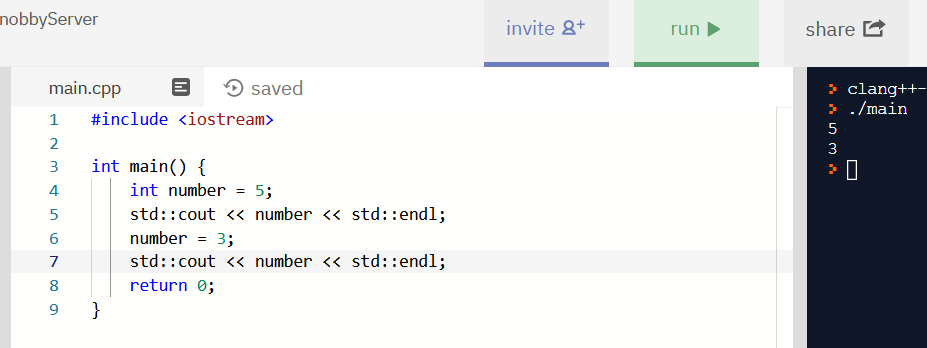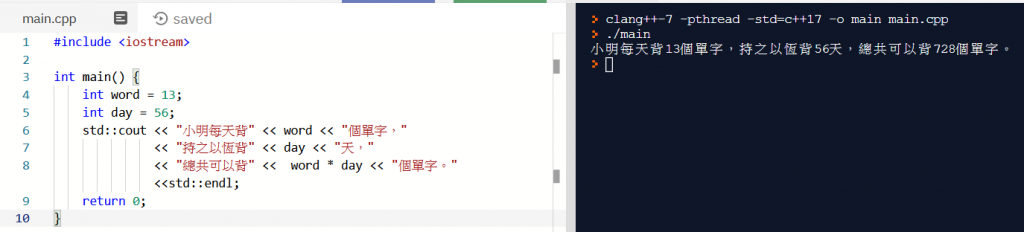# 【从零开始的c世界生活】(2) 基础变数宣告、添加注解的方式

(建议可以直接使用线上写程式工具repl.it，省去初学安装的麻烦)

# 快速複习一下

``````#include <iostream>

int main() {
std::cout << "Hello, World!"<< std::endl;
std::cout << "Hello, 小马!"<< std::endl;
return 0;
}
``````

`#include`在c++中是引入函式库的意思，

`cout`是函式库`<iostream>`里面的指令，

`cout`是输出的意思，`std::`表示`cout`这个函数来自`std`这个命名空间

# 变数宣告

``````#include <iostream>

int main() {
int number = 5;
return 0;
}
``````

`int`是integer(整数)的意思，

``````#include <iostream>

int main() {
int number = 5;
number = 3;
return 0;
}
``````

``````#include <iostream>

int main() {
int number = 5;
std::cout << number << std::endl;
number = 3;
std::cout << number << std::endl;
return 0;
}
``````

`5`
`3`(结果会印在右边的黑色视窗中)

## 建议: 在宣告变数的同时就初始化

``````#include <iostream>

int main() {
int number;
std::cout << number << std::endl;
number = 3;
std::cout << number << std::endl;
return 0;
}
``````

`number`的值是未定义的，

# 基础四则运算

## 範例: 用功的小明

(当解决的问题很複杂时，也不是按计算机能解决的)

``````#include <iostream>

int main() {
int word = 13;
int day = 56;
std::cout << "小明每天背" << word << "个单字，"
<< "持之以恆背" << day << "天，"
<< "总共可以背" <<  word * day << "个单字。" <<std::endl;
return 0;
}
``````# 注解- 让你的程式更好读

`/* */`是多行注解，可以把注解的内容以`/* */`包起来
`//` 是一行注解，把注解的内容写在`//`后面

``````#include <iostream>

/*

*/

int main() {
int word = 13; //每天背的单字量
int day = 56; //持续背单字的天数
std::cout << "小明每天背" << word << "个单字，"
<< "持之以恆背" << day << "天，"
<< "总共可以背" <<  word * day << "个单字。" <<std::endl;
return 0;
}
``````

# 附录: 合法的变数名称

• 变数名称只能用英文字母、数字、_(底线)或中文字组成 (但建议不要用中文字帮变数取名)
• 英文字大小写不一样，例如: Name 和 name 是不同的变数名称
• c++内建关键字不可以当作变数名称 (例如: int, for)
• 变数不可以用阿拉伯数字当开头，如 3a 是不合法的名字

# 课后理解小测试

``````#include <iostream>

int main() {
int word = 13; //每天背的单字量
int day = 56; //持续背单字的天数
std::cout << "小明每天背" << word << "个单字，"; //如果这行跟下一行加分号可以吗?
<< "持之以恆背" << day << "天，";
<< "总共可以背" <<  word * day << "个单字。" <<std::endl;
return 0;
}
``````• * 没有更多文章了

1. 技术原理